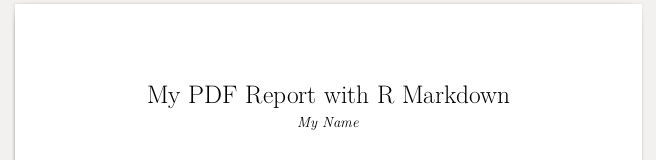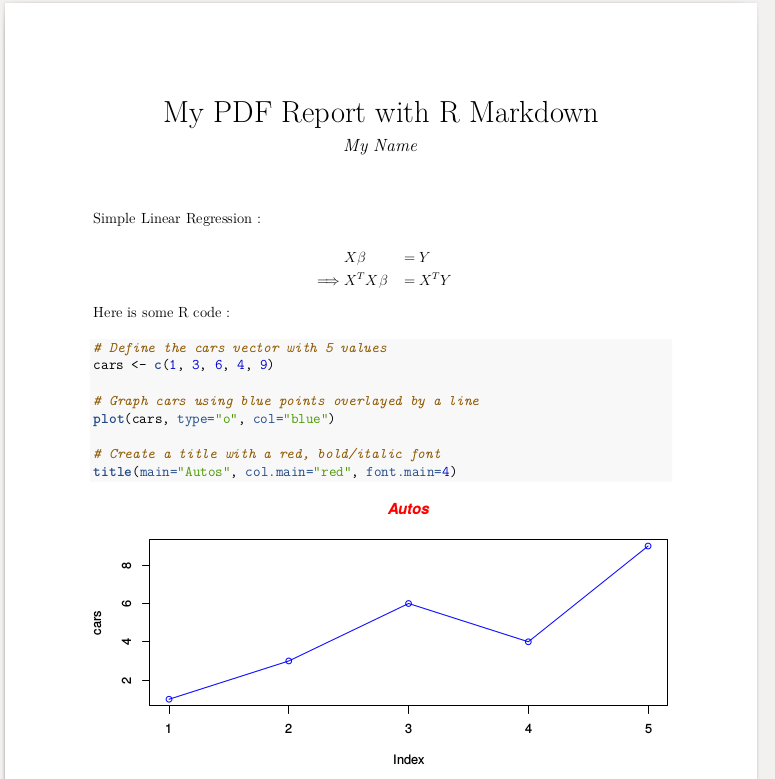# Creating an R Markdown PDF output (command line version)

## Introduction

Today, I will be talking about how to generate a nice pdf report with text, code, plots, and formulas using R markdown.

For those of you who are in a hurry, you will find the entire code at the end of this post. Simply skip to Step 2 to learn how to convert it into pdf.

Once again, I will explain how to do this in command line. Because why would anyone need graphical interfaces when they have Vim ?

## Step 1 : Create a basic .Rmd file

Save the following lines in a file named, say, “my_report.Rmd” :

---
title: "My PDF Report with R Markdown"
author:
- First Name, Last Name
output: pdf_document
fontsize: 12pt
---


## Step 2 : Convert .Rmd -> PDF

Command line in the same directory

>> Rscript -e “rmarkdown::render(‘./my_report.Rmd’)”

You sould find a file named my_report.pdf in the same directory.

The file should look like this :## Step 3 : Add some text, and a formula

Simple Linear Regression :

\begin{aligned} &X \beta &&= Y \\ \implies &X^{T} X \beta &&= X^{T} Y \\ \end{aligned}


Step 2 to update the PDF

Formulas are written using LaTex formatting.

## Step 4 : Add some R code, and a plot

Here is some R code :

{r}
# Define the cars vector with 5 values
cars <- c(1, 3, 6, 4, 9)

# Graph cars using blue points overlayed by a line
plot(cars, type="o", col="blue")

# Create a title with a red, bold/italic font
title(main="Autos", col.main="red", font.main=4)



Step 2 to update the PDF

More “generic” plot ideas here.

## Step 5 : Change the plot size

In the above code, change

{r}

for

{r, fig.width=8, fig.height=4}


Step 2 to update the PDF

## Step 5 : Summary

Here is the entire sample code and the resulting PDF you can expect to have.

---
title: "My PDF Report with R Markdown"
author:
- My Name
output: pdf_document
fontsize: 12pt
---

Simple Linear Regression :

\begin{aligned} &X \beta &&= Y \\ \implies &X^{T} X \beta &&= X^{T} Y \\ \end{aligned}

Here is some R code :

{r, fig.width=8, fig.height=4}
# Define the cars vector with 5 values
cars <- c(1, 3, 6, 4, 9)

# Graph cars using blue points overlayed by a line
plot(cars, type="o", col="blue")

# Create a title with a red, bold/italic font
title(main="Autos", col.main="red", font.main=4)
`